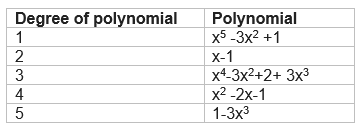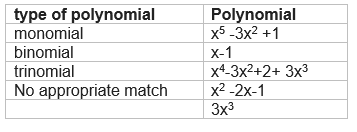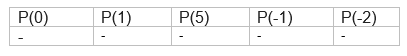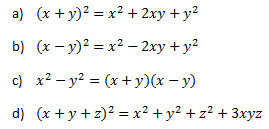# class 9 polynomials extra questions

Here we are trying to give the class 9 polynomials extra questions and polynomials class 9 important questions It tests the basic concepts and at the same makes the student comfortable with the questions

## Match the column

A.B.## Table Type

P(x)=5x2 -3x+7## True or False statement

1. P(x) =x-1 and g(x) =x2-2x +1 . p(x) is a factor of g(x)
2. The factor of 3x2 –x-4 are (x+1)(3x-4)
3. Every linear polynomial has only one zero
4. Every real number is the zero’s of zero polynomial
5. A binomial may have degree 4
6. 0,2 are the zeroes of x2-2x
7. The degree of zero polynomial is not defined

Question 1
Factorize following
1. x2 +9x+18
2. 3x3 –x2-3x+1
3. x3-23x2+142x-120
4. 1+8x3

## Multiple choice Questions

Question 1
Find the remainder when x4+x3-2x2+x+1 is divided by x-1 (a)1
(b)5
(c)2
(d)3

Question 2
Which of these identities is not true?Question 3
If $\frac {x}{y} + \frac {y}{x}=-1$ and ($x y, \ne 0$ ) , the value of $x^3 – y^3$ is
(a) 1
(b) -1
(c) 0
(d) 2

Question 4
If $p+q+r=0$ ,then the value of $\frac {p^2}{qr} + \frac {q^2}{pr} + \frac {r^2}{pq}$ is
(a) 1
(b) 3
(c) -1
(d) 0

## class 9 polynomials important questions

Question 1
Find the value of $47^3 - 30^3 - 17^3$

Question 2
Simply following
(i) $\frac {(p^2 -q^2)^3 + (q^2 -r^2)^3 + (r^2 -p^2)^3}{(p -q)^3 + (q -r)^3 + (r -p)^3}$
(ii) $(x - y)^3+ (y – z)^3+ (z - x)^3$

Question 3
Find the values of a and b if $x^3 -ax^2 -13x +b=0$ has (x-1) and (x+3) as factors

## Summary

This Polynomials class 9 extra questions is prepared keeping in mind the latest syllabus of CBSE . This has been designed in a way to improve the academic performance of the students. If you find mistakes , please do provide the feedback on the mail. You can download this test as pdf also as below